18 Geocomputation
 In:=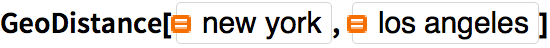Out=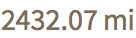Plot New York and Los Angeles on a map:
 In:=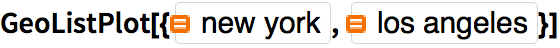Out=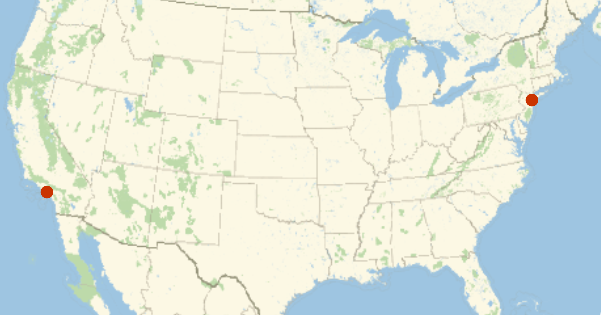In:=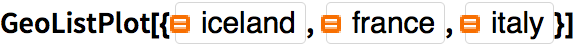Out=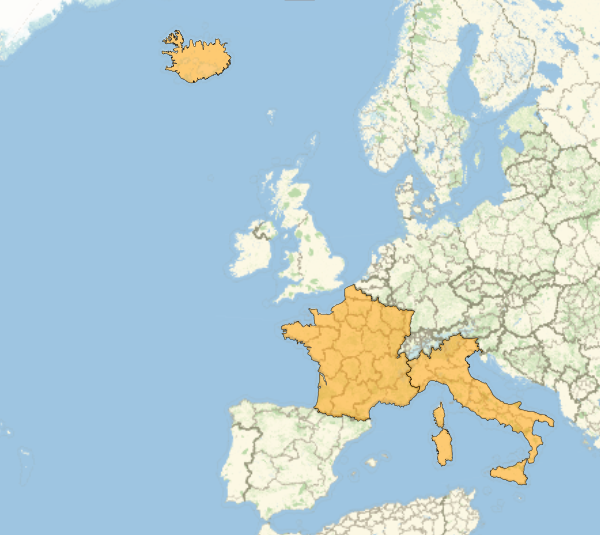In:=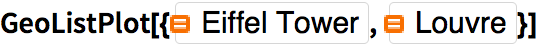Out=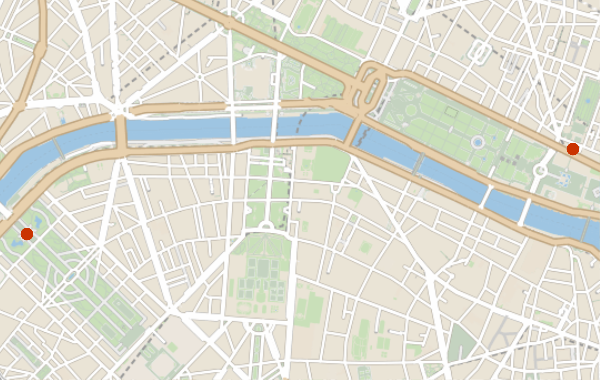GeoListPlot is the analog for geography of ListPlot. GeoGraphics is the analog of Graphics.
 In:=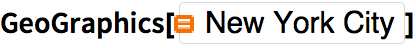Out=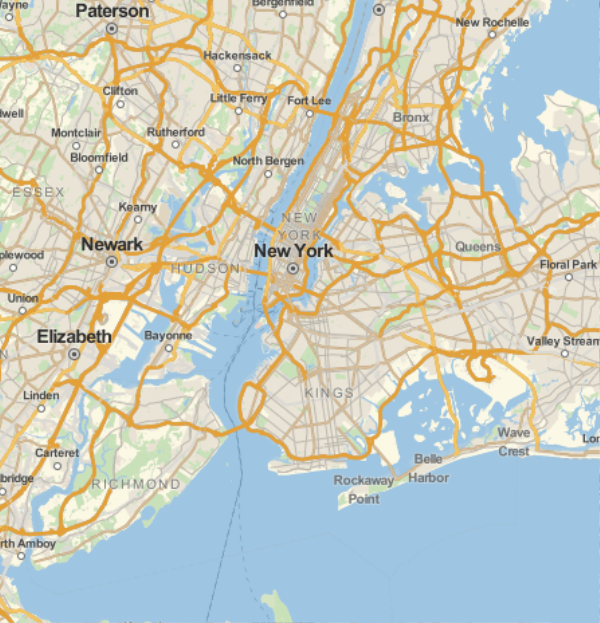GeoPath represents a path on the surface of the Earth.
 In:=Out=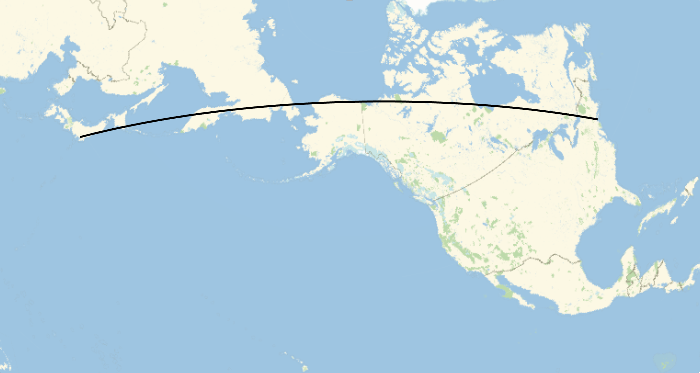In:=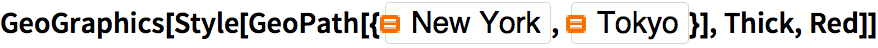Out=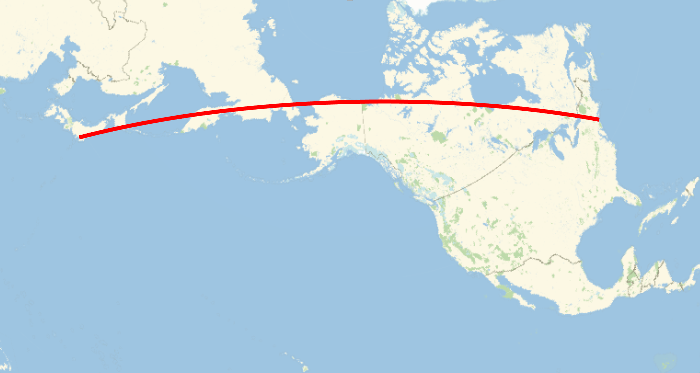GeoDisk is the analog of Disk; you specify its center and its radius.
Show a 1-mile-radius disk around the Eiffel Tower:
 In:=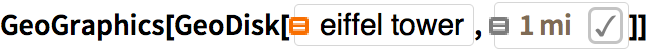Out=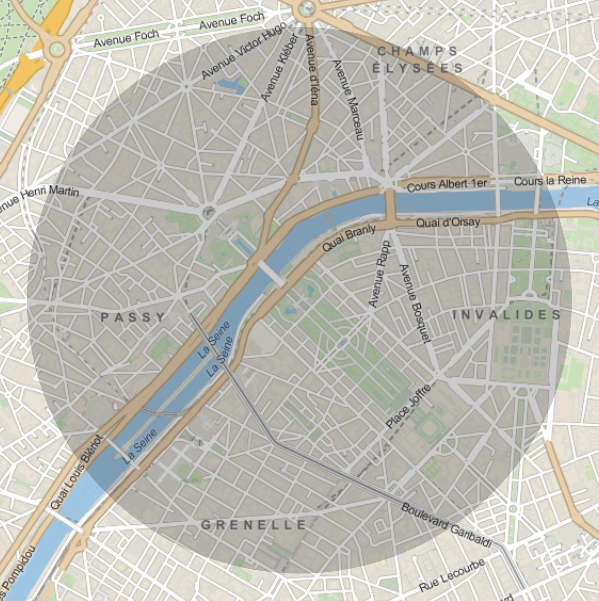In:=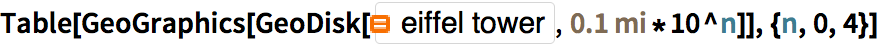Out=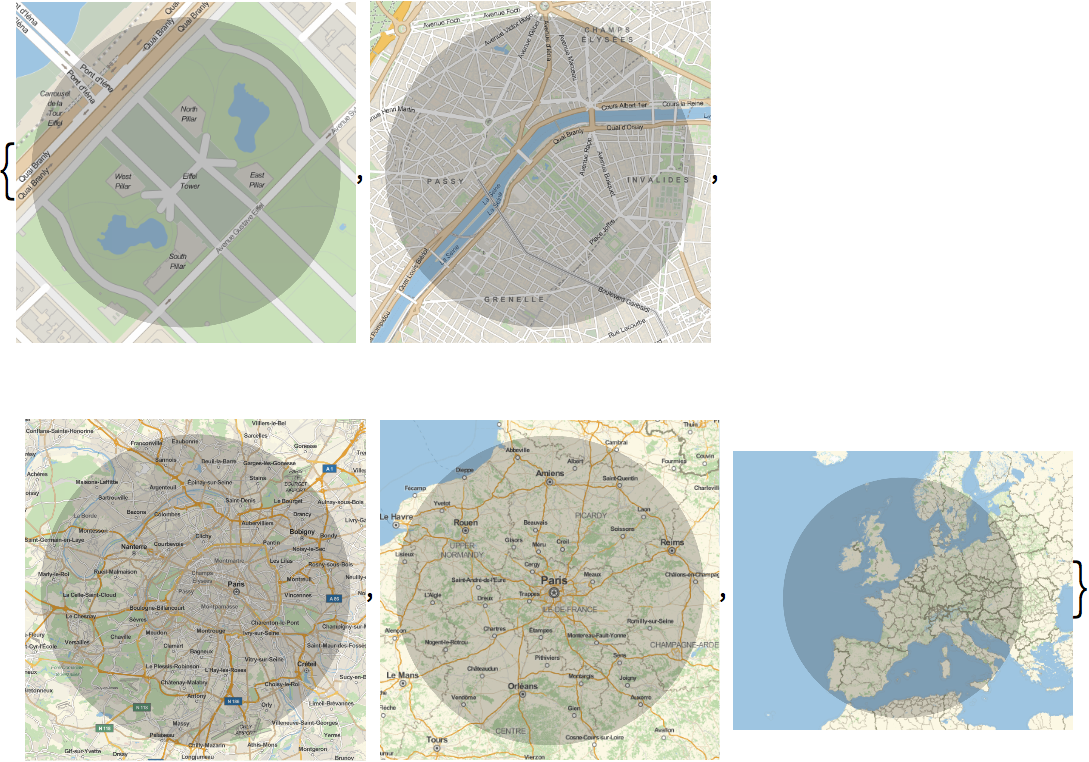GeoPosition gives a position on the Earth. The numbers it contains are longitude and latitudethe standard coordinates on the surface of the Earth.
Find the geo position of the Eiffel Tower:
 In:=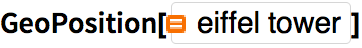Out=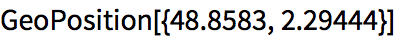Draw a 4000-mile-radius disk around 0 latitude, 0 longitude:
 In:=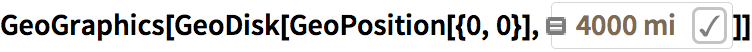Out=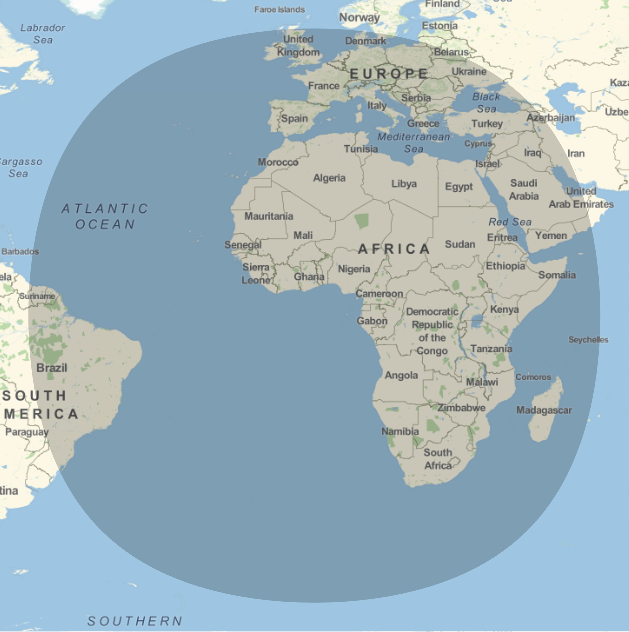GeoNearest finds whats nearest to a given place on the Earth. You tell it what type of thing to look for, and how many you want it to find.
Find the nearest 5 countries to 0 longitude, 0 latitude:
 In:=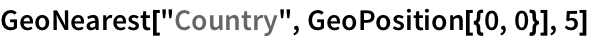Out=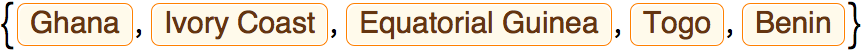In:=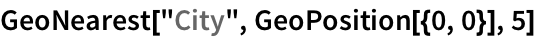Out=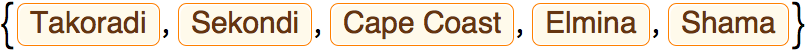Find where your computer (or phone, etc.) thinks it is:
 In:=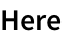Out=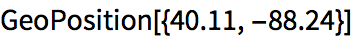You can do computations with Here.
Compute how far it is to the Eiffel Tower:
 In:=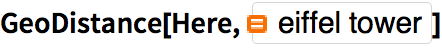Out=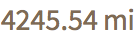In:=Out=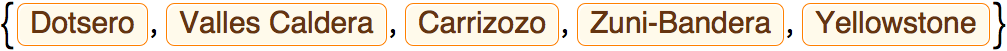Plot volcanoes on a map:
 In:=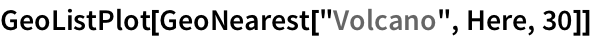Out=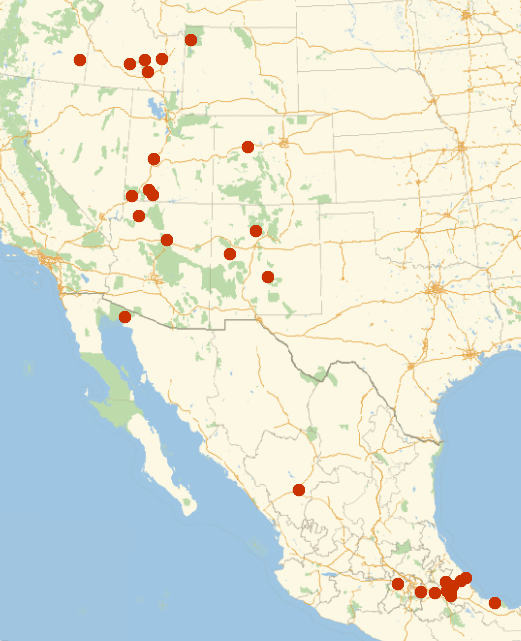GeoDistance[entity1,entity2] geo distance between entities GeoListPlot[{entity1,entity2, ...}] plot a list of entities on a map GeoGraphics[...] map constructed from primitives GeoPath[{entity1,entity2}] path between entities GeoDisk[entity,r] disk with radius r around an entity Here where your computer, phone, etc. thinks it is GeoPosition[entity] geo position of an entity GeoNearest["type",location,n] nearest n objects of a certain type to a location
18.1Find the distance from New York to London. »
Expected output:
 Out[]=Answer & check your solution
18.2Divide the distance from New York to London by the distance from New York to San Francisco. »
Expected output:
 Out[]=Answer & check your solution
18.3Find the distance from Sydney to Moscow in kilometers. »
Expected output:
 Out[]=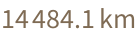Answer & check your solution
18.4Generate a map of the United States. »
Expected output:
 Out[]=Answer & check your solution
18.5Plot on a map Brazil, Russia, India and China. »
Expected output:
 Out[]=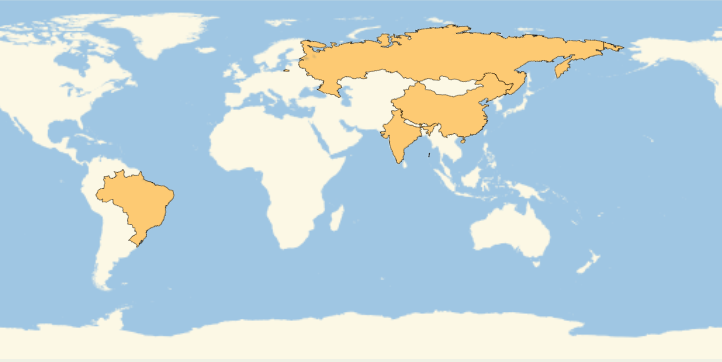Answer & check your solution
18.6Plot on a map the path from New York City to Beijing. »
Expected output:
 Out[]=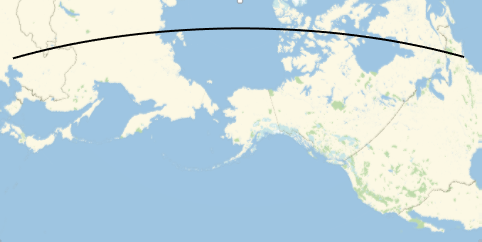Answer & check your solution
18.7Plot a disk centered on the Great Pyramid, with radius 10 miles. »
Expected output:
 Out[]=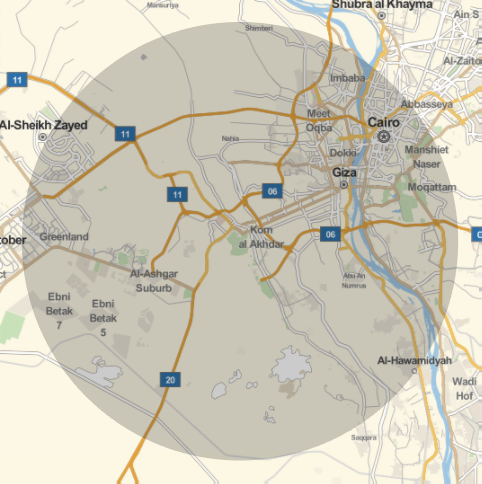Answer & check your solution
18.8Plot a disk centered on New York with a radius large enough to just reach San Francisco. »
Expected output:
 Out[]=Answer & check your solution
18.9Find the nearest 5 countries to the North Pole (GeoPosition["NorthPole"]). »
Expected output:
 Out[]=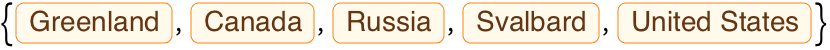Answer & check your solution
18.10Find the flags of the 3 countries nearest to latitude 45°, longitude 0°»
Expected output:
 Out[]=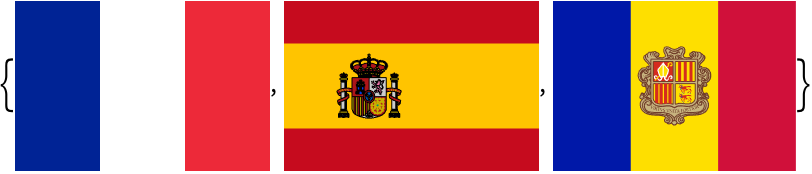Answer & check your solution
18.11Plot the 25 volcanoes closest to Rome. »
Expected output:
 Out[]=Answer & check your solution
18.12Find the difference in latitude between New York and Los Angeles. »
Expected output:
 Out[]=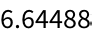Answer & check your solution
+18.1Plot on a map the countries in NATO. »
Expected output:
 Out[]=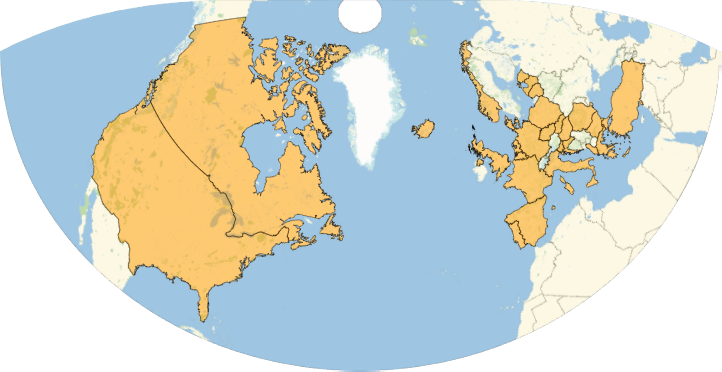Answer & check your solution
+18.2Plot on a map a thick red line from Moscow to Beijing and a thick blue line from Washington, DC to London. »
Expected output:
 Out[]=Answer & check your solution
+18.3Find the distance from 0 latitude, 0 longitude to the Eiffel Tower. »
Expected output:
 Out[]=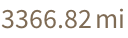Answer & check your solution
+18.4Plot a disk styled red of radius 100 miles centered on Los Angeles. »
Expected output:
 Out[]=Answer & check your solution
+18.5Make a list of plots showing disks with radii 1, 2 and 3 miles around the Empire State Building. »
Expected output:
 Out[]=Answer & check your solution
+18.6Find the 5 countries nearest to New York City. »
Expected output:
 Out[]=Answer & check your solution
+18.7Find the nearest ocean to Chicago. »
Expected output:
 Out[]=Answer & check your solution
Yes. Just use the GeoProjection option. There are more than 300 built-in projections to choose from. The default projection used in any particular case depends on the scale and location of the map.
It uses the function FindGeoLocation. On a mobile device, this will normally ask for your GPS position. On a computer, itll normally try to deduce your location from your internet addressthis wont always work correctly. You can always explicitly set your geo location by assigning a value to \$GeoLocation.
Use the option GeoRangedistance (e.g. GeoRange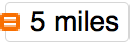) or GeoRangeplace (e.g. GeoRange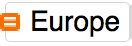), as discussed in Section 20.
Yes. Use TravelDirections. GeoDistance gives the direct shortest path; TravelDistance gives the path following roads, etc. TravelTime gives estimated travel time.